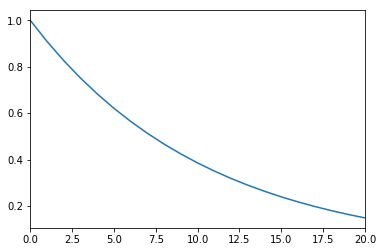# Net Present Value (NPV) - Examples¶

In :
import pandas as pd

%matplotlib inline


## PV Example¶

In :
lifetime = 20 #years
discount_rate = 0.04 #0.0775 #per unit
size = 100 #kW
specific_cost = 800 #EUR/kW
fom = 20 #EUR/kW/a
fit = 0.1 #EUR/kWh
flh = 1000 #h/a
flows["total_flow"] = flows.sum(axis=1)
flows["discount_factor"] = [(1+discount_rate)**(-t) for t in range(lifetime+1)]
flows["discounted_total_flow"] = flows["total_flow"]*flows["discount_factor"]

In :
flows.head()

Out:
investment FOM income total_flow discount_factor discounted_total_flow
0 -80000 0 0.0 -80000.0 1.000000 -80000.000000
1 0 -2000 10000.0 8000.0 0.961538 7692.307692
2 0 -2000 10000.0 8000.0 0.924556 7396.449704
3 0 -2000 10000.0 8000.0 0.888996 7111.970869
4 0 -2000 10000.0 8000.0 0.854804 6838.433528
In :
flows.sum()

Out:
investment               -80000.000000
FOM                      -40000.000000
income                   200000.000000
total_flow                80000.000000
discount_factor              14.590326
discounted_total_flow     28722.610760
dtype: float64
In :
(size*specific_cost/(sum(flows["discount_factor"])-1)+2500)/1e5

Out:
0.6650050203417118
In :
flows["discount_factor"].plot()

Out:
<matplotlib.axes._subplots.AxesSubplot at 0x7f4c3e060f28>## Nuclear Example¶

In :
lifetime = 40 #years
discount_rate = 0.10 #per unit
size = 3e6 #kW
specific_cost = 5000 #EUR/kW
decommissioning_cost = 0 #EUR/kW
fom = 20 #EUR/kW/a
fuel = 10 #EUR/MWh
market_value = 50 #EUR/MWh
flh = 8000 #h/a
flows["investment"] = [-size*specific_cost] + *(lifetime-1) + [-size*decommissioning_cost]
flows["total_flow"] = flows.sum(axis=1)
flows["discount_factor"] = [(1+discount_rate)**(-t) for t in range(lifetime+1)]
flows["discounted_total_flow"] = flows["total_flow"]*flows["discount_factor"]

In :
flows.head()

Out:
investment FOM income total_flow discount_factor discounted_total_flow
0 -1.500000e+10 0.0 0.000000e+00 -1.500000e+10 1.000000 -1.500000e+10
1 0.000000e+00 -60000000.0 1.200000e+09 1.140000e+09 0.909091 1.036364e+09
2 0.000000e+00 -60000000.0 1.200000e+09 1.140000e+09 0.826446 9.421488e+08
3 0.000000e+00 -60000000.0 1.200000e+09 1.140000e+09 0.751315 8.564989e+08
4 0.000000e+00 -60000000.0 1.200000e+09 1.140000e+09 0.683013 7.786353e+08
In :
flows.sum()

Out:
investment              -1.500000e+10
FOM                     -2.400000e+09
income                   4.800000e+10
total_flow               3.060000e+10
discount_factor          1.077905e+01
discounted_total_flow   -3.851882e+09
dtype: float64
In [ ]:


In [ ]: